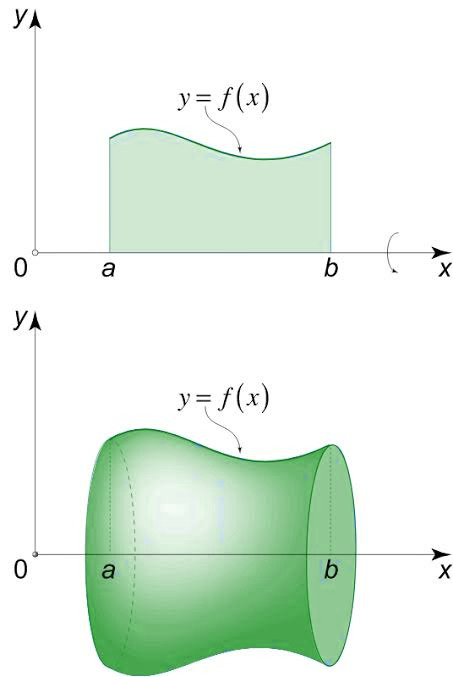# Volume of solid of revolution

A solid of revolution is generated by revolving a plane area R about a line L known as axis of revolution in the plane. Below image shows an example of solid of revolution.We shall calculate the volume of solid of revolution when the equation of the curve is given in parametric form and polar form.

1. Parametric Form :
If the equation of curve in parametric form is given by:

x= f(t) and y= g(t)

Where t varies from t1to t2, then the volume of revolution:2. Polar form:
Given the equation of curve in polar form as r=f(θ), where θ varies from θ1 to θ2, the volume of revolution is calculated using the given formulas:

• About the initial line OX i.e., x-axis (θ=0) –• About the line perpendicular to the initial line i.e. along OY (θ=π/2) –Let us see the following examples.

Example-1:
Determine the volume of solid of revolution generated by revolving the curve whose parametric equations are –

X= 2t+3 and y= 4t2-9

About x-axis for t= -3/2 to 3/2.

Explanation :
We know that volume of solid revolved about x-axis when equation is in parametric form is given byUsing this value we getExample-2:
Find the volume of solid generated by revolving curve r= 2a cos θ about the initial line OX.

Explanation :
We know that volume of solid generated by revolving about OX in when equation is in polar form is given byAlso for OX, θ=0
SoPutUsing these values we getAttention reader! Don’t stop learning now. Get hold of all the important CS Theory concepts for SDE interviews with the CS Theory Course at a student-friendly price and become industry ready.

My Personal Notes arrow_drop_upCheck out this Author's contributed articles.

If you like GeeksforGeeks and would like to contribute, you can also write an article using contribute.geeksforgeeks.org or mail your article to contribute@geeksforgeeks.org. See your article appearing on the GeeksforGeeks main page and help other Geeks.

Please Improve this article if you find anything incorrect by clicking on the "Improve Article" button below.

Article Tags :

1

Please write to us at contribute@geeksforgeeks.org to report any issue with the above content.